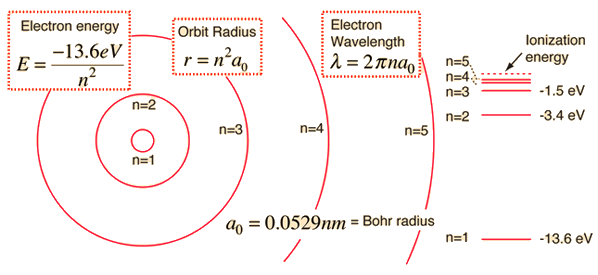# How do you calculate the energy of an electron in the ground state of a hydrogen atom ?

Sep 27, 2015

You can calculate the ground state energy using The Bohr Model

#### Explanation:

A simple expression for the energy of an electron in the hydrogen atom is:

$E = - \frac{13.6}{{n}^{2}}$ where the energy is in electron volts

$n$ is the principle quantum number.This gives rise to the familiar electron energy level diagram where they converge and coalesce.

So for an electron in $n = 1$:

$E = - 13.6 \text{eV}$

To convert to joules you can x this by $1.6 \times {10}^{- 19}$

For many - electron atoms quite complicated approximate methods can be used which take into account factors such as electron interactions and screening effects.